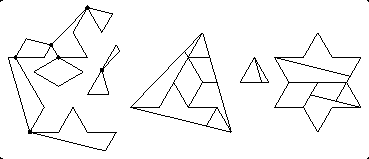# Hinged Dissections

## Group Members

Math Consultant: Emily Clark
Designer: Nathan Glass

## Project Idea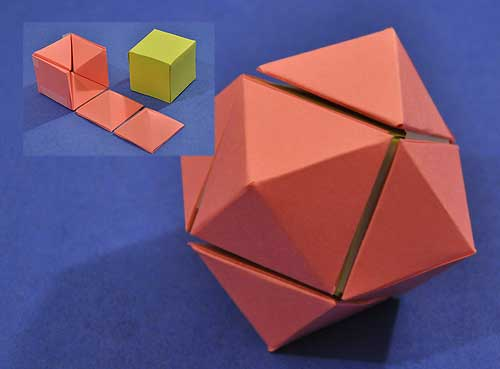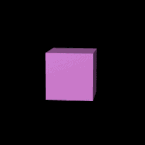For our project we will be exploring the rhombic dodecahedron which is a 3D shape that is made when you turn a 3D cube inside out.  Our method for printing this will be printing out 6 pyramids of the same dimension and taping them together so that we can form both a cube as well as the rhombic dodecahedron.  According to page 217 of Things to Make and Do in the Fourth Dimension, the rhombic dodecahedron is a polyhedron with diamond-shaped faces, but it is not a Platonic solid.  It is closely related to the 4-dimensional hyper-diamond, which is the 4D hyper cube turned inside out.

## First Steps

We started our project by first looking at the rhombic dodecahedron discussed in Chapter 10 on page 217.  It has 12 faces, 24 edges, and 14 vertices.  We also came up with a plan on how we are going to go about printing our design.  We figured out that in order to make the separate pyramids we print fit well together, we need to make base of each pyramid a square, meaning that their dimensions are the same number, and the height should be half of the dimensions of the base. We have also made a design of our print on Tinkercad.  Lastly, we made a paper version of what our print will look like: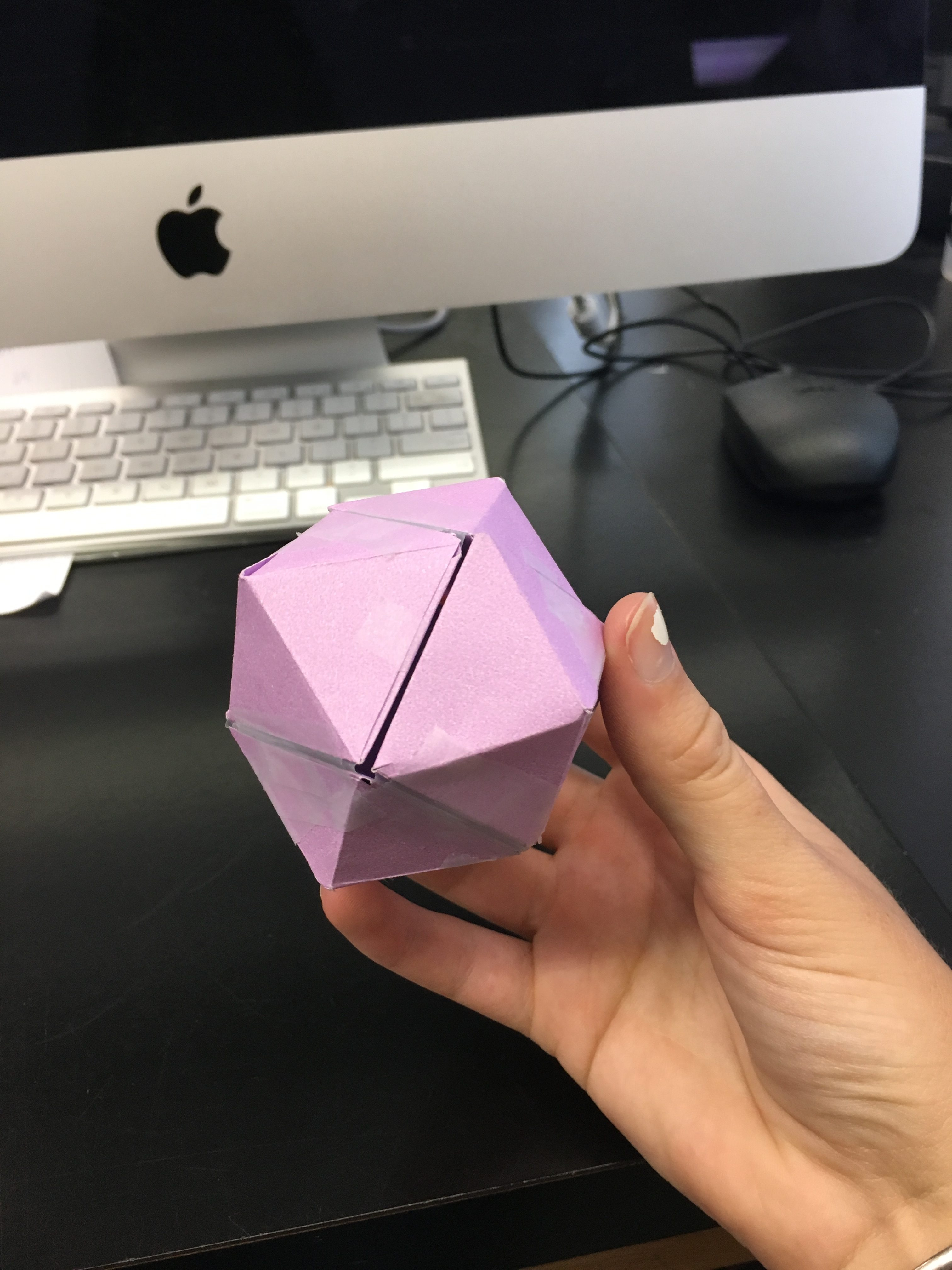Looking at the rhombic dodecahedron, and seeing how it could be turned inside out to become a square, we became interested in this type of relationship, and discovered it could be found in other shapes and dimensions as well, and looked into the concepts of dual polyhedra and hinged dissections.

## Design Process

First we printed pyramids and taped them together so that they could form a cube and a rhombic dodecahedron.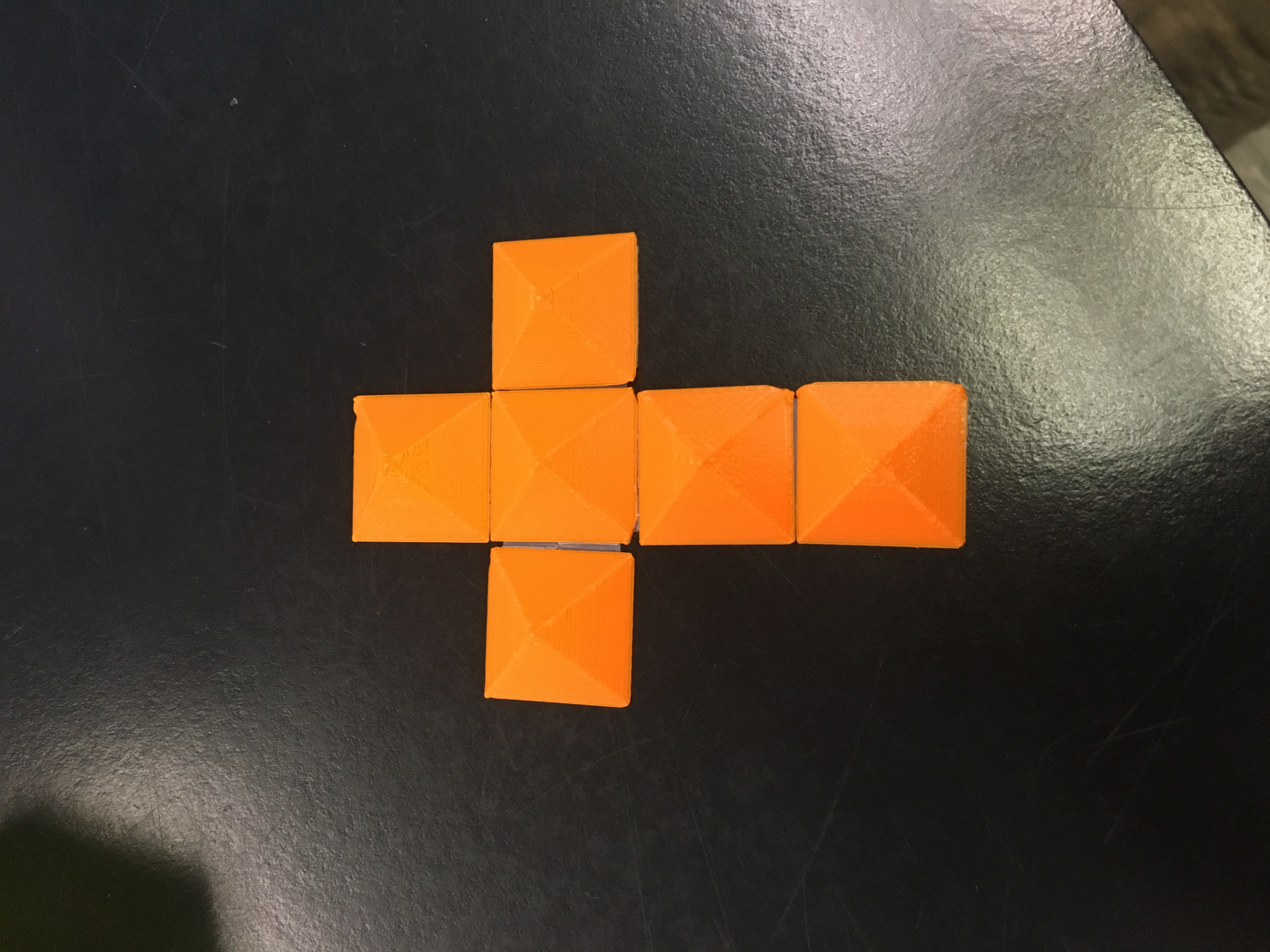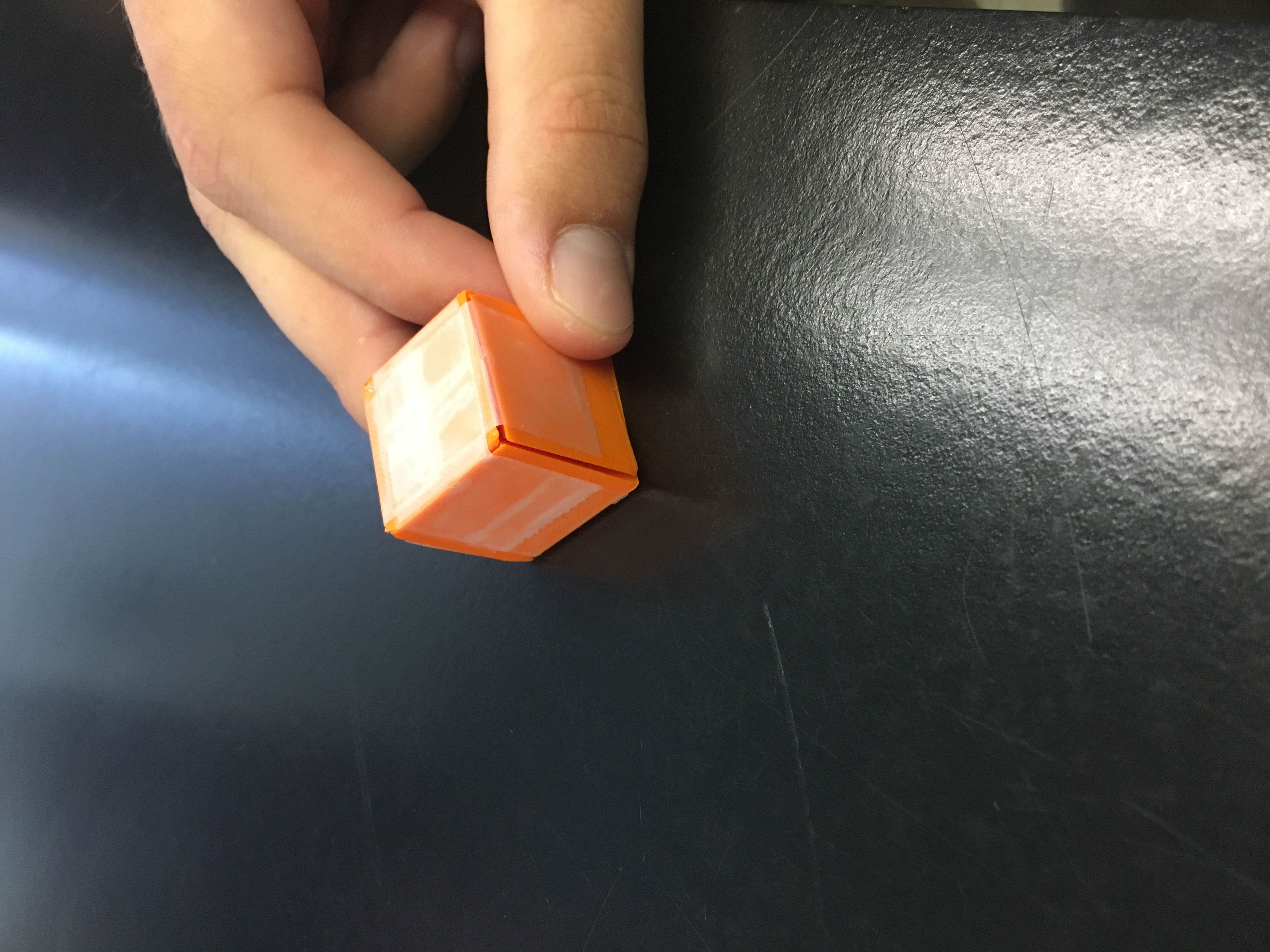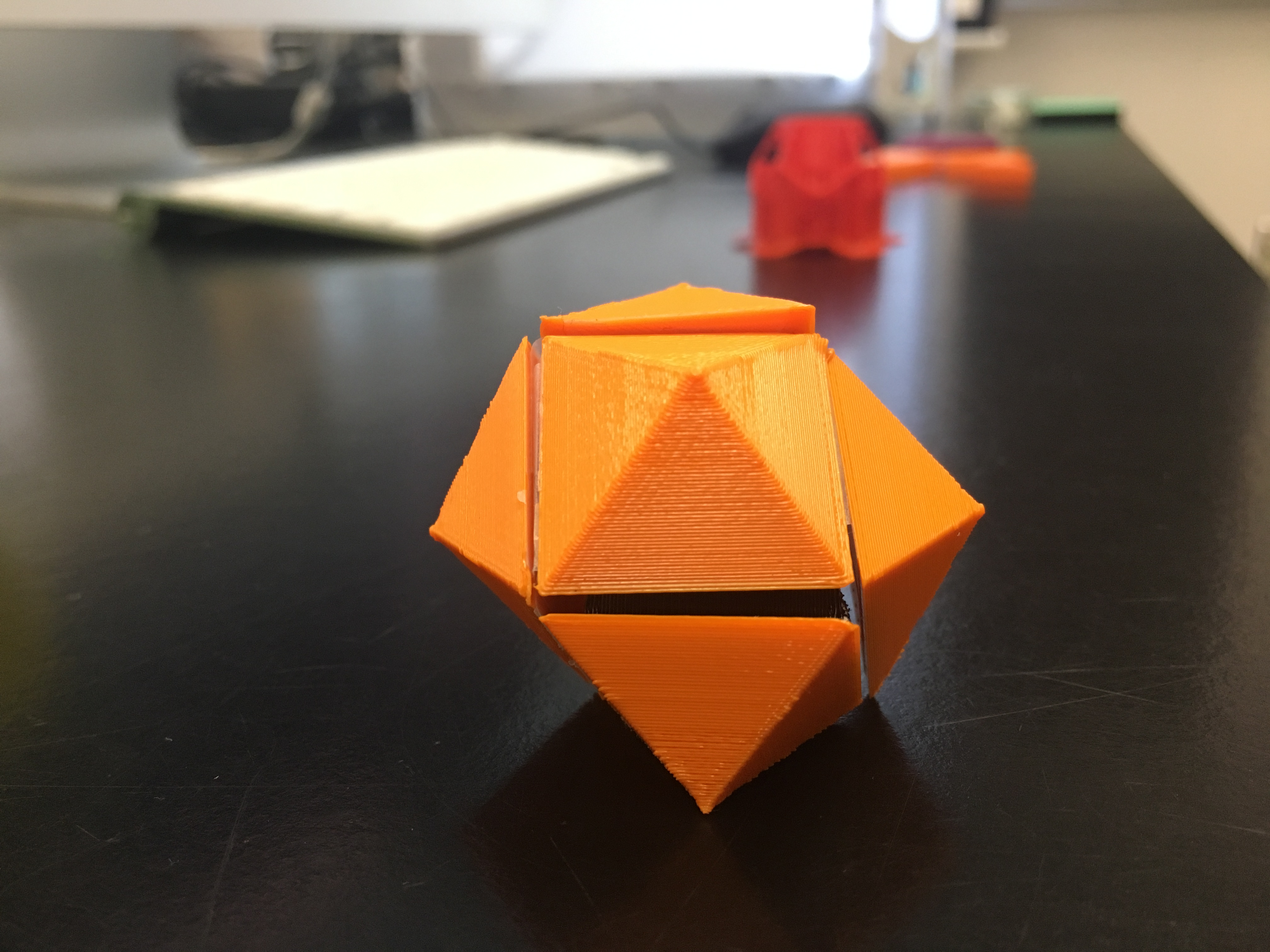Next we tried to print an exoskeleton of a dodecahedron but we could not get the supports off without breaking the actual print.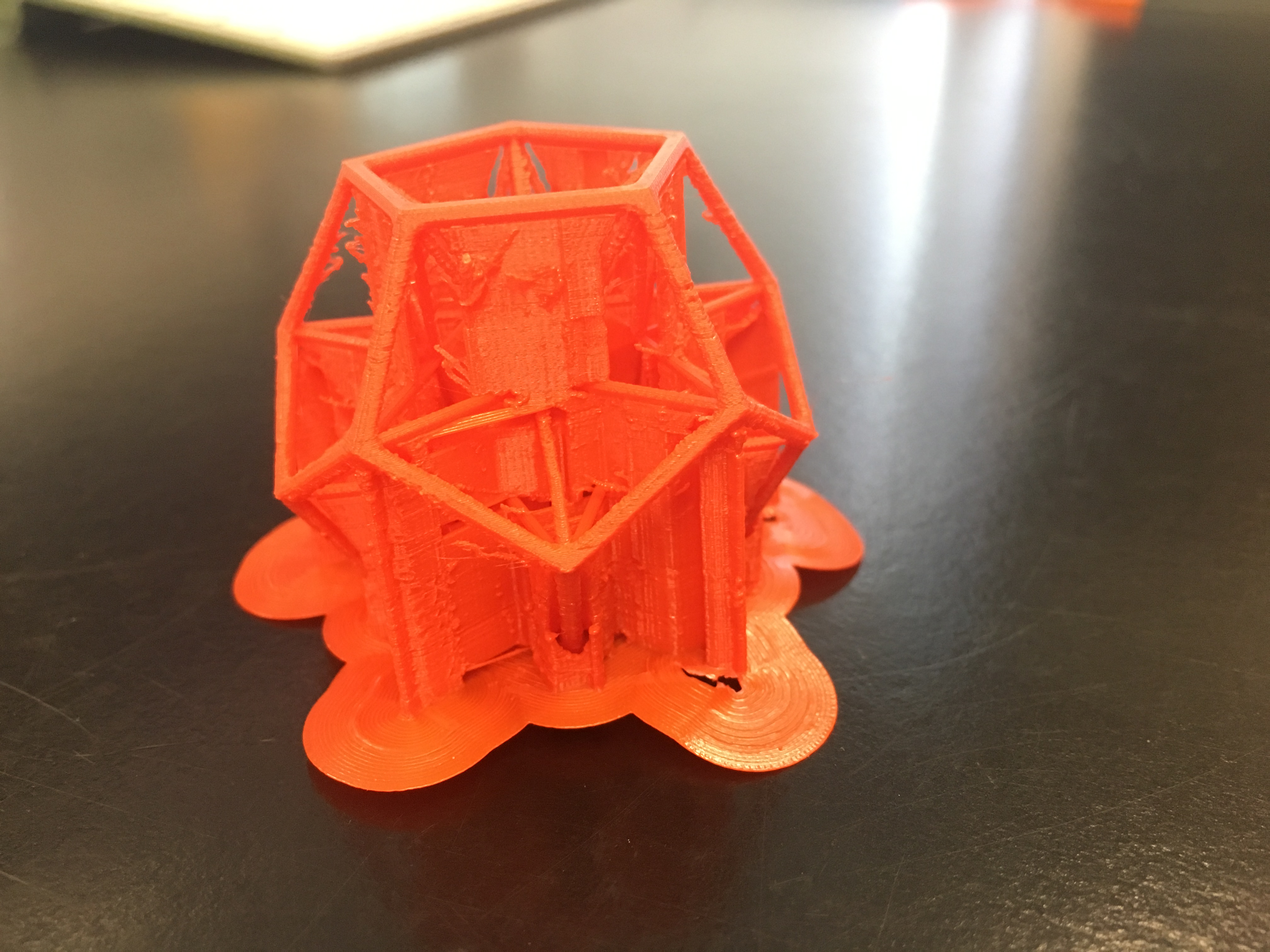We also printed out the pieces that make up a hinged dissection from a triangle to a square.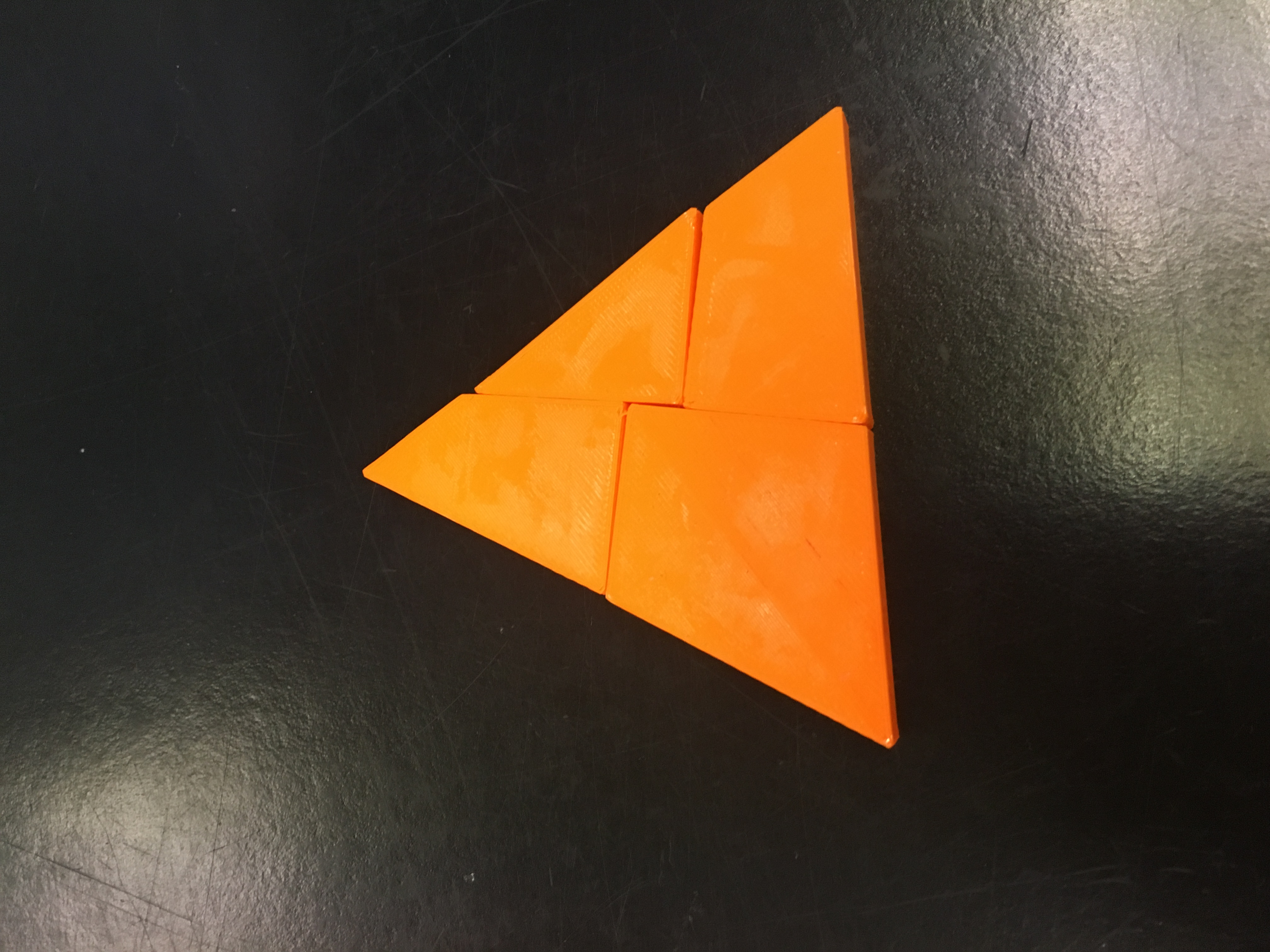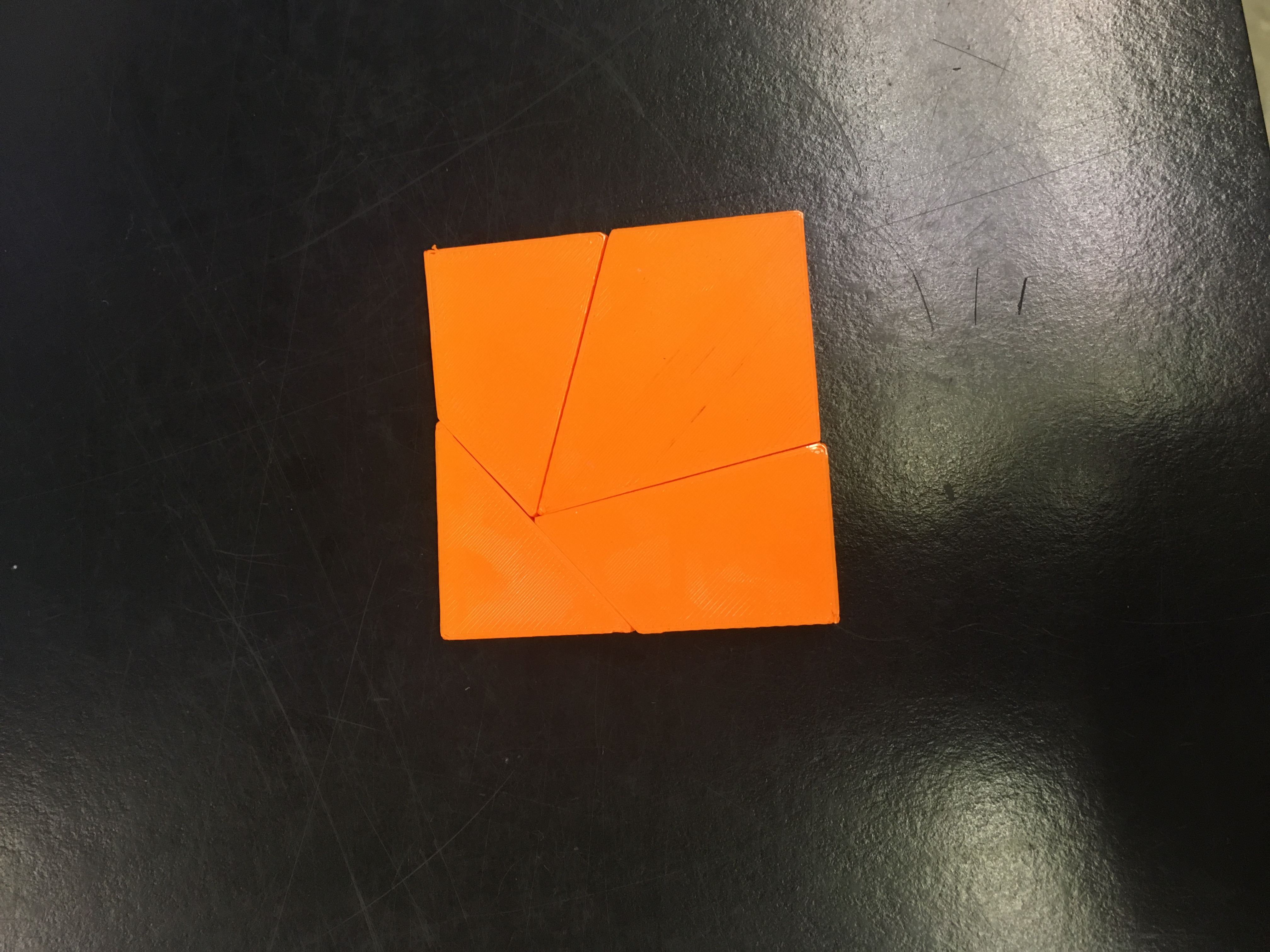## Final Prints of Hinged Dissections

Hinged rhombic dodecahedron to cubeTriangle to square hinged dissection## Mathematics

### Dual Polyhedron

A polyhedron is a solid in 3D with flat polygonal faces, straight edges, and sharp corners or vertices.  Every polyhedron is associated with a second figure, where the vertices of one correspond to the faces of the other, and the edges between pairs of vertices of one polyhedron correspond to the edges between pairs of faces to the other.  These two polyhedron that share this relationship are called dual polyhedron.  Starting with any regular polyhedron, the dual can be constructed by placing a point in the center of each face of the original, connecting each point with the new points on each of the neighboring faces, and then erasing the original polyhedron.  An example of this relationship can be seen between the dodecahedron and the icosahedron.  The centers of the faces of the dodecahedron are the vertices of the icosahedron, and the faces of the icosahedron are the vertices of the dodecahedron.

Originally we thought that dual polyhedron were closely related to hinge dissections, but after looking further into it concluded that they are not as closely related as we originally thought.Another example of this relationship is seen with the cube and the rhombic dodecahedron.

## Presentation

We started this project by looking at the rhombic dodecahedron discussed in Chapter 10 on page 217, which is a dual polyhedron to an Archimedean solid.  A dual polyhedron is any polyhedron that is associated with a second figure, where the vertices of one correspond to the faces of the other, and the edges between pairs of vertices of one polyhedron correspond to the edges between pairs of faces to the other.  There are 13 Archimedean solids, which were first discovered by the Greek mathematician Archimedes.  Archimedean solids are composed of regular polygons meeting in identical vertices, meaning that for any two vertices, there is a global isometry (mapping) of the entire solid that takes one vertex to the other.We made a print of the rhombic dodecahedron out of six pyramids, and were intrigued by the fact that with all of the pyramids being attached, we could form both the rhombic dodecahedron and a cube.  We decided to look into relationships similar to this.  First we explored dual polyhedrons, but found it was it wasn’t quite what we were looking for.  Next we looked into hinge dissections and found that this relationship was similar to the cube and rhombic dodecahedron.

### Hinge DissectionsIn 1807, it was proved that two polygons with an equal area must share a common dissection.  A hundred years later, in 1907, Henry Dudeney introduced the square-to-triangle hinge dissection.  In a hinged dissection, a shape is disassembled into smaller pieces so that each smaller piece in connected by one edge in a chain, and then rotated to make a new shape with the same area.  In 2007, Erik Demaine proved that there is always a hinged dissection between two equal-area polygons.  The hinge dissection can be thought of as the 2D equivalent to the 3D concept of dual polyhedron, or the relationship seen between the cube and rhombic dodecahedron.